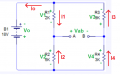# KVL orientation question

#### WBahn

Joined Mar 31, 2012
28,498
The assignment of voltages and currents is completely arbitrary -- you don't even have to abide by the passive sign convention.

Consider the following choices of assignments, all of which were made via flipping a coin:Let's write KVL equations for the outer loop, the leftmost loop, and the top loop on the right (in that order), going clockwise starting from the lower left corner of each loop and summing up the voltage gains:

Loop #1: - V0 + V3 - V4 = 0
Loop #2: - V0 - V1 - V2 = 0
Loop #3: + V1 + V3 + Vab = 0

We could write several more (four, I think) loop equations, but they would all be linear combinations of these three.

Using Ohm's Law, we can relate the voltages to the currents as follows:

V1 = I1·R1
V2 = - I2·R2
V3 = I3·R3
V4 = - I4·R4

Applying KCL at the upper three nodes, summing the current into the node:

Top Node: - I0 - I1 + I3 = 0
Node A: I1 + I2 = 0
Node B: - I3 + I4 = 0

We could write another node equation for the bottom node, but it would be a linear combination of these three.

Now it is a matter of plug and chug:

Vab = - V1 - V3
Vab = - I1·R1 - I3·R3

V0 = -18 V
I2 = - I1
I4 = I3

From Loop #1
- V0 + V3 - V4 = 0
- V0 + I3·R3 - I4·R4 = 0
- V0 + I3·R3 + I3·R4 = 0
I3 = V0/(R3 + R4)

From Loop #2:
- V0 - V1 - V2 = 0
- V0 - I1·R1 + I2·R2
- V0 - I1·R1 - I1·R2
I1 = - V0/(R1+R2)

Therefore
Vab = - I1·R1 - I3·R3
Vab = - [- V0/(R1+R2)]·R1 - [V0/(R3 + R4)]·R3
Vab = V0·{[R1/(R1+R2)] - [R3/(R3 + R4)]}

Substituting in the values for this particular circuit:

Vab = (-18 V)·{[(2 kΩ)/(2 kΩ + 6kΩ)] - [9 kΩ/(9 kΩ + 3 kΩ)]}
Vab = (-18 V)·{[2 kΩ/8 kΩ] - [9 kΩ/12 kΩ]}
Vab = (-18 V)·{(1/4) - (3/4)}
Vab = (-18 V)·(-1/2)
Vab = 9 V

Note that your original answer of Vab = -9V is wrong. You calculated Vba. Vab, by convention, is Va - Vb.

#### Transatlantic

Joined Feb 6, 2014
44
But how did you solve for VR1 and VR2 ??
I did that with voltage divider equations as in the first post

Also did your solution for VR1 is consistent with your assumed current direction ? The answer is NO.
Because if the current is flow from point A toward upper R1 terminal VR1 is negative (-4.5V) therefore
Vab = -Vr3 - Vr1 = 13.5V - (-4.5V) = -9V
Thank you. I think I see now.

So would I be correct in thinking that you can only use arbitrary current directions and polarities if you're computing everything consistently using KCL and KVL from the result of linear combinations (as per WBahns post)? and that my mistake was I computed it partially using other means (voltages dividers) and then tried to apply the arbitrary directions and polarities with those results.

#### WBahn

Joined Mar 31, 2012
28,498
Regardless of how you do it, you must be completely, totally, one hundred percent consistent with how you have chosen to do it. You can't just throw formulas at your work blindly.